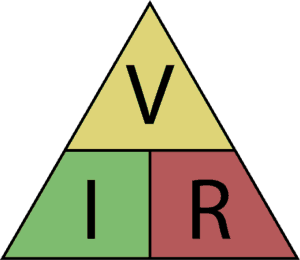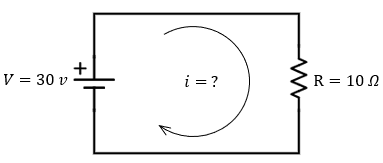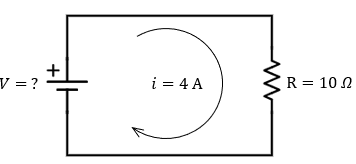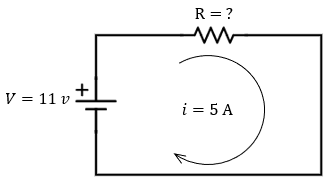# Ohm's Law Examples with Definition Formula, and Ohm's TriangleOhm's law is a mathematical formula that describes the relationship between voltage, current, and resistance in an electrical circuit.

The name of the law is after the German physicist Georg Simon Ohm.

Nevertheless, the reader must consider that not all conductors work under the ohm's law since some conductors are called non-ohmic, whose resistance value varies depending on the voltage applied.

## Ohm's Law Definition

Ohm's law states that "the force of a direct current in an electrical conductor is directly proportional to the potential difference and inversely proportional to the conductor's specific resistance."

## Ohm's Law Formula

In mathematical terms, Ohm's law is applied by the equation:Where:

• “R” is the electrical resistance. By resistance, we mean the obstacle that the current encounters in its path, for example, a light bulb. The higher the resistance is, the more difficult it will be for the current to cross the section. The unit of measurement for resistance is ohms, symbolized by the Greek letter omega (Ω).

• “I” is the intensity of an electric current (the amperage) that passes through a cross-sectional conductor expelled in Amperes (A).

• “V” is the voltage; it is the electrical energy with which a battery or other electrical device drives electrons through the circuit. The power is more significant when the voltage is higher; therefore, the electrons flow faster, increasing the electric current. The tension or voltage is measured in Volts (V).

As we saw in ohm's law definition, the amperage is directly proportional to the applied voltage and inversely proportional to the resistance. If the voltage drops, the amperage drops proportionally.

For the voltage, on the other hand, the higher it is, the greater the attractive force it generates to move the charges, so for the same resistive value, it will be directly proportional to the current.

### Ohm's Law for Alternating Current (AC)

For the analysis of alternating current circuits, Resistance (R) is replaced by Impedance (Z), which considers the phase shifts between voltage and amperage and the effects of electromagnetic fields produced in the electronic circuit components. But the most normal thing in basic electronics is to analyze circuits in direct current or apply simplifications that allow us to study them as they were.

### Ohm's Law and Electrical Power

Sometimes the formula for electrical power is used by applying Ohm's law.

The power formula is as follows (with the unit of watts):

P = V·I, (power = voltage x current)

and its variants: V = P / I and I = P / V,

The two variants can be substituted in Ohm's law formula. For example, if we start from the formula to calculate the voltage and we substitute, we have the following formula:

V = (P / V) R

Isolating the power, we have the following form:

V² · R = P

## What Is Ohm's Law Triangle?

Ohm's law triangle is a trick for remembering the formula.To obtain the triangle formula, we have to cover the variable we want to get with our hands. If the remaining elements are on top of the other, they are divided. If they remain in line, we jas have to multiply the values.

The three possible combinations are:

• I = V / R

• V = I·R

• R = V / I

## Ohm's Law Example

For a better understanding of  Ohm's law, we will turn to a simple hydraulic example:

Let's imagine a hose connected to the tank of a fire truck.In this example, the elements equivalent to an electrical circuit are the following:

• Voltage (V). The voltage equals the power of the truck's water pump. The water pump “applies a voltage” to the circuit as if it were a battery in an electrical circuit.

• Resistance (R). The resistance of the electrical circuit is equivalent to the resistance offered by the hose (circle's area). If the hose has a large diameter, the water will flow more easily than if the diameter is small. In the example of the electrical circuit, the concept of resistance is equivalent but is expressed in Ohms (Ω).

• Amperage (I): In our example, amperage is the number of water molecules that flow through a section of hose per unit of time. In an electrical circuit, the equivalent of molecules is electrical charges.

In this simile, it is easy to understand that if we increase the pump's capacity (voltage), the water flow (amperage) will increase. In the same way, if we use a smaller hose (greater resistance), the flow will also decrease.

## Examples of Ohm's Law Practice Problems

Let's move on to solve some numerical problems of Ohm's law:

### Example 1 of Ohm's Law Problem

Find the amperage of the current that feeds a toy washing machine with a resistance of 10 ohms and works with a battery with a potential difference of 30 V.Solution: To solve this problem, it is enough to return to the data of the problem, which in this case would be the resistance of 10 Ohms, and a voltage of 30 Volts, so we would have.

R=10Ω

V=10v

I=?

The problem asks us for the current, so we must apply ohm's law to find it.

I=V·R=30v10Ω=3A

The final conclusion is that we need three Amps to power the toy washing machine. As we can see, the amperage is proportional to the voltage.

### Example 2 of Ohm's Law Problem

Find the voltage between two points of the circuit, through which a current of 4 amps passes and presents a resistance of 10 ohms.Solution: In the same way as the previous example, what we need is to return to our data, which in this case would be the four amps that cross the iron circuit and the 10-ohm resistor, so:

I=4A

R=10Ω

V=?

In this case, our formula will be the same, only now we will clear it.

I=V·R

v=I⋅R

Now, what we have to do is just replace our data in the ohm's law formula.

V=(4A)⋅(10Ω)=40v

So we would have 40 Volts as a response, which would be the ones that cross between the two points of the plate.

Before we go any further with two more problems, there is something important to mention

Current is a flow of electrons that travels from one point to another, so the more resistance a material has, the lower the amount of current that passes over it.

### Example 3 of Ohm's Law Problem

Calculate the resistance crossed by a current with an amperage of 5 amps and a potential difference of 11 volts.Solution:  If we always consider the data of our problems, it is easier to solve a physics problem. In this case, we would have the following:

I=5A

v=11V

R=?

Now from ohm's law, we isolate the value of R in order to obtain our final equation:

R=V·I

R=11·5A=2.2Ω

So our resistance would be 2.2 Ohms, which would end our exercise.

Author:

Published: September 13, 2021
Last review: September 13, 2021